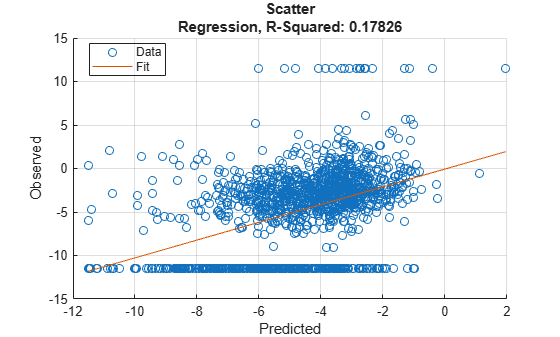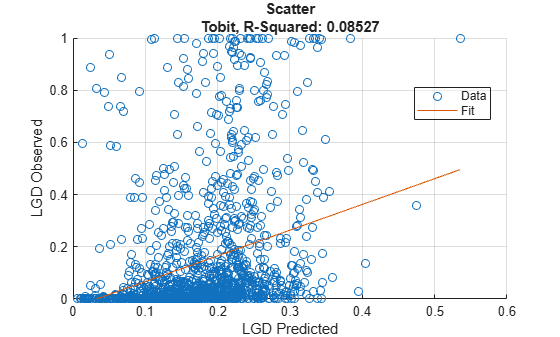Main Content

# modelAccuracy

Compute R-square, RMSE, correlation, and sample mean error of predicted and observed LGDs

## Syntax

``AccMeasure = modelAccuracy(lgdModel,data)``
``[AccMeasure,AccData] = modelAccuracy(___,Name,Value)``

## Description

example

````AccMeasure = modelAccuracy(lgdModel,data)` computes the R-square, root mean square error (RMSE), correlation, and sample mean error of observed vs. predicted loss given default (LGD) data. `modelAccuracy` supports comparison against a reference model and also supports different correlation types. By default, `modelAccuracy` computes the metrics in the LGD scale. You can use the `ModelLevel` name-value pair argument to compute metrics using the underlying model's transformed scale.```

example

````[AccMeasure,AccData] = modelAccuracy(___,Name,Value)` specifies options using one or more name-value pair arguments in addition to the input arguments in the previous syntax.```

## Examples

collapse all

This example shows how to use `fitLGDModel` to fit data with a `Regression` model and then use `modelAccuracy` to compute the R-Square, RMSE, correlation, and sample mean error of predicted and observed LGDs.

Load Data

Load the loss given default data.

```load LGDData.mat head(data)```
```ans=8×4 table LTV Age Type LGD _______ _______ ___________ _________ 0.89101 0.39716 residential 0.032659 0.70176 2.0939 residential 0.43564 0.72078 2.7948 residential 0.0064766 0.37013 1.237 residential 0.007947 0.36492 2.5818 residential 0 0.796 1.5957 residential 0.14572 0.60203 1.1599 residential 0.025688 0.92005 0.50253 investment 0.063182 ```

Partition Data

Separate the data into training and test partitions.

```rng('default'); % for reproducibility NumObs = height(data); c = cvpartition(NumObs,'HoldOut',0.4); TrainingInd = training(c); TestInd = test(c);```

Create `Regression` LGD Model

Use `fitLGDModel` to create a `Regression` model using training data.

```lgdModel = fitLGDModel(data(TrainingInd,:),'regression'); disp(lgdModel) ```
``` Regression with properties: ResponseTransform: "logit" BoundaryTolerance: 1.0000e-05 ModelID: "Regression" Description: "" UnderlyingModel: [1x1 classreg.regr.CompactLinearModel] PredictorVars: ["LTV" "Age" "Type"] ResponseVar: "LGD" ```

Display the underlying model.

`disp(lgdModel.UnderlyingModel)`
```Compact linear regression model: LGD_logit ~ 1 + LTV + Age + Type Estimated Coefficients: Estimate SE tStat pValue ________ ________ _______ __________ (Intercept) -4.7549 0.36041 -13.193 3.0997e-38 LTV 2.8565 0.41777 6.8377 1.0531e-11 Age -1.5397 0.085716 -17.963 3.3172e-67 Type_investment 1.4358 0.2475 5.8012 7.587e-09 Number of observations: 2093, Error degrees of freedom: 2089 Root Mean Squared Error: 4.24 R-squared: 0.206, Adjusted R-Squared: 0.205 F-statistic vs. constant model: 181, p-value = 2.42e-104 ```

Compute R-Square, RMSE, Correlation, and Sample Mean Error of Predicted and Observed LGDs

Use `modelAccuracy` to compute the `RSquared`, `RMSE`, `Correlation`, and `SampleMeanError` of the predicted and observed LGDs for the test data set.

`[AccMeasure,AccData] = modelAccuracy(lgdModel,data(TestInd,:))`
```AccMeasure=1×4 table RSquared RMSE Correlation SampleMeanError ________ _______ ___________ _______________ Regression 0.070867 0.25988 0.26621 0.10759 ```
```AccData=1394×3 table Observed Predicted_Regression Residuals_Regression _________ ____________________ ____________________ 0.0064766 0.00091169 0.0055649 0.007947 0.0036758 0.0042713 0.063182 0.18774 -0.12456 0 0.0010877 -0.0010877 0.10904 0.011213 0.097823 0 0.041992 -0.041992 0.89463 0.052947 0.84168 0 3.7188e-06 -3.7188e-06 0.072437 0.0090124 0.063425 0.036006 0.023928 0.012078 0 0.0034833 -0.0034833 0.39549 0.0065253 0.38896 0.057675 0.071956 -0.014281 0.014439 0.0061499 0.008289 0 0.0012183 -0.0012183 0 0.0019828 -0.0019828 ⋮ ```

Generate a scatter plot of predicted and observed LGDs using `modelAccuracyPlot`.

`modelAccuracyPlot(lgdModel,data(TestInd,:),'ModelLevel',"underlying")`This example shows how to use `fitLGDModel` to fit data with a `Tobit` model and then use `modelAccuracy` to compute R-Square, RMSE, correlation, and sample mean error of predicted and observed LGDs.

Load Data

Load the loss given default data.

```load LGDData.mat head(data)```
```ans=8×4 table LTV Age Type LGD _______ _______ ___________ _________ 0.89101 0.39716 residential 0.032659 0.70176 2.0939 residential 0.43564 0.72078 2.7948 residential 0.0064766 0.37013 1.237 residential 0.007947 0.36492 2.5818 residential 0 0.796 1.5957 residential 0.14572 0.60203 1.1599 residential 0.025688 0.92005 0.50253 investment 0.063182 ```

Partition Data

Separate the data into training and test partitions.

```rng('default'); % for reproducibility NumObs = height(data); c = cvpartition(NumObs,'HoldOut',0.4); TrainingInd = training(c); TestInd = test(c);```

Create `Tobit` LGD Model

Use `fitLGDModel` to create a `Tobit` model using training data.

```lgdModel = fitLGDModel(data(TrainingInd,:),'tobit'); disp(lgdModel) ```
``` Tobit with properties: CensoringSide: "both" LeftLimit: 0 RightLimit: 1 ModelID: "Tobit" Description: "" UnderlyingModel: [1x1 risk.internal.credit.TobitModel] PredictorVars: ["LTV" "Age" "Type"] ResponseVar: "LGD" ```

Display the underlying model.

`disp(lgdModel.UnderlyingModel)`
```Tobit regression model: LGD = max(0,min(Y*,1)) Y* ~ 1 + LTV + Age + Type Estimated coefficients: Estimate SE tStat pValue _________ _________ _______ __________ (Intercept) 0.058257 0.027276 2.1358 0.032809 LTV 0.20126 0.031373 6.415 1.7363e-10 Age -0.095407 0.0072543 -13.152 0 Type_investment 0.10208 0.018054 5.6542 1.7802e-08 (Sigma) 0.29288 0.005704 51.346 0 Number of observations: 2093 Number of left-censored observations: 547 Number of uncensored observations: 1521 Number of right-censored observations: 25 Log-likelihood: -698.383 ```

Compute R-Square, RMSE, Correlation, and Sample Mean Error of Predicted and Observed LGDs

Use `modelAccuracy` to compute `RSquared`, `RMSE`, `Correlation`, and `SampleMeanError` of predicted and observed LGDs for the test data set.

`[AccMeasure,AccData] = modelAccuracy(lgdModel,data(TestInd,:),'CorrelationType',"kendall")`
```AccMeasure=1×4 table RSquared RMSE Correlation SampleMeanError ________ _______ ___________ _______________ Tobit 0.08527 0.23712 0.29964 -0.034412 ```
```AccData=1394×3 table Observed Predicted_Tobit Residuals_Tobit _________ _______________ _______________ 0.0064766 0.087889 -0.081412 0.007947 0.12432 -0.11638 0.063182 0.32043 -0.25724 0 0.093354 -0.093354 0.10904 0.16718 -0.058144 0 0.22382 -0.22382 0.89463 0.23695 0.65768 0 0.010234 -0.010234 0.072437 0.1592 -0.086761 0.036006 0.19893 -0.16292 0 0.12764 -0.12764 0.39549 0.14568 0.2498 0.057675 0.26181 -0.20413 0.014439 0.14483 -0.13039 0 0.094123 -0.094123 0 0.10944 -0.10944 ⋮ ```

Generate a scatter plot of the predicted and observed LGDs using `modelAccuracyPlot`.

`modelAccuracyPlot(lgdModel,data(TestInd,:))`## Input Arguments

collapse all

Loss given default model, specified as a previously created `Regression` or `Tobit` object using `fitLGDModel`.

Data Types: `object`

Data, specified as a `NumRows`-by-`NumCols` table with predictor and response values. The variable names and data types must be consistent with the underlying model.

Data Types: `table`

### Name-Value Pair Arguments

Specify optional comma-separated pairs of `Name,Value` arguments. `Name` is the argument name and `Value` is the corresponding value. `Name` must appear inside quotes. You can specify several name and value pair arguments in any order as `Name1,Value1,...,NameN,ValueN`.

Example: ```[AccMeasure,AccData] = modelAccuracy(lgdModel,data(TestInd,:),'DataID','Testing','CorrelationType','spearman')```

Correlation type, specified as the comma-separated pair consisting of `'CorrelationType'` and a character vector or string.

Data Types: `char` | `string`

Data set identifier, specified as the comma-separated pair consisting of `'DataID'` and a character vector or string. The `DataID` is included in the output for reporting purposes.

Data Types: `char` | `string`

Model level, specified as the comma-separated pair consisting of `'ModelLevel'` and a character vector or string.

• `'top'` — The accuracy metrics are computed in the LGD scale at the top model level.

• `'underlying'` — For a `Regression` model only, the metrics are computed in the underlying model's transformed scale. The metrics are computed on the transformed LGD data.

Note

`ModelLevel` has no effect for a `Tobit` model because there is no response transformation.

Data Types: `char` | `string`

LGD values predicted for `data` by the reference model, specified as the comma-separated pair consisting of `'ReferenceID'` and a `NumRows`-by-`1` numeric vector. The `modelAccuracy` output information is reported for both the `lgdModel` object and the reference model.

Data Types: `double`

Identifier for the reference model, specified as the comma-separated pair consisting of `'ReferenceID'` and a character vector or string. `'ReferenceID'` is used in the `modelAccuracy` output for reporting purposes.

Data Types: `char` | `string`

## Output Arguments

collapse all

Accuracy measure, returned as a table with columns `'RSquared'`, `'RMSE'`, `'Correlation'`, and `'SampleMeanError'`. `AccMeasure` has one row if only the `lgdModel` accuracy is measured and it has two rows if reference model information is given. The row names of `AccMeasure` report the model ID and data ID (if provided).

Accuracy data, returned as a table with observed LGD values, predicted LGD values, and residuals (observed minus predicted). Additional columns for predicted and residual values are included for the reference model, if provided. The `ModelID` and `ReferenceID` labels are appended in the column names.

## More About

collapse all

### Model Accuracy

Model accuracy measures the accuracy of the predicted probability of LGD values using different metrics.

• R-squared — To compute the R-squared metric, `modelAccuracy` fits a linear regression of the observed LGD values against the predicted LGD values

`$LG{D}_{obs}=a+b\ast LG{D}_{pred}+\epsilon$`

The R-square of this regression is reported. For more information, see Coefficient of Determination (R-Squared).

• RMSE — To compute the root mean square error (RMSE), `modelAccuracy` uses the following formula where N is the number of observations:

`$RMSE=\sqrt{\frac{1}{N}{\sum }_{i=1}^{N}\left(LG{D}_{i}^{obs}-LG{D}_{i}^{pred}{\right)}^{2}}$`

• Correlation — This is the correlation between the observed and predicted LGD:

`$corr\left(LG{D}_{obs},LG{D}_{pred}\right)$`

For more information and details about the different correlation types, see `corr`.

• Sample mean error — This is the difference between the mean observed LGD and the mean predicted LGD or, equivalently, the mean of the residuals:

`$SampleMeanError=\frac{1}{N}{\sum }_{i=1}^{N}\left(LG{D}_{i}^{obs}-LG{D}_{i}^{pred}\right)$`

 Baesens, Bart, Daniel Roesch, and Harald Scheule. Credit Risk Analytics: Measurement Techniques, Applications, and Examples in SAS. Wiley, 2016.

 Bellini, Tiziano. IFRS 9 and CECL Credit Risk Modelling and Validation: A Practical Guide with Examples Worked in R and SAS. San Diego, CA: Elsevier, 2019.

## Support

#### Effective Model Risk Management with MATLAB

Download white paper